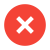﻿ Temperature Difference - Delta T - Performance Monitoring Watertreatment

# Temperature Difference – Delta T (ΔT)

The term Delta T (ΔT) is in science, the difference of temperature between two measuring points. The temperature differs either in time and/or position. We at Merus use it for example, to measure the efficiency of a heat exchanger. Or for checking the performance of a heating system or a cooling system.

Δ,  (Delta) is the fourth letter of the Greek alphabet. Whereas Δ is the symbol of the capital letter and δ is the lower case letter. The Δ is also used as a mathematical symbol. Δ describes the "difference" of any changeable quantity. In a process ΔT is a value to show the difference between two measured temperatures.

Differential temperature is expressed in Kelvin. No matter if the value is measured in °Celsius or in Fahrenheit. Therefore the values can be compared easily. The units are expressed in Kelvin(K) without using any degree symbol. The lowest temperature is 0 K. No negative numbers exist in the Kelvin scale.

### The equation of delta t is: ΔT = T2 - T1To the left there is the drawing of a tubular heat exchanger. There is the cooling water entering at point B and leaving warmer at point D.  And there is the liquid stream to be cooled, entering point C and getting out at point A. To get a usable ΔT, one should compare the same liquid going in and out. The entrance temperature in the heat exchanger at B would be T1. And the outlet from the heat exchanger coming out at D is T2.

The cooling water entering the heat exchanger will get warmer on its way through the exchanger. The difference is then the ΔT. If T1 and T2 is monitored regularly, one can see the perfomance of the cooling at the delta T. Monitoring this temperature over a longer time, will give indication about the grade of fouling in the heat exchanger.

In pipes differential temperature usually describes the difference between the necessary pipe temperature (Tp) and the expected lowest ambient temperature (Ta). So

ΔT = Tp - Ta

Having all 4 temperatures and 1 flow rate of a heat exchanger, one is able to calculate the Heat Transfer Coefficient.

In a heating or a cooling system in a building the delta T is used to compare the cool water send in the house and the warmer water which comes back. So one can see if and how much the Air Condition is used.